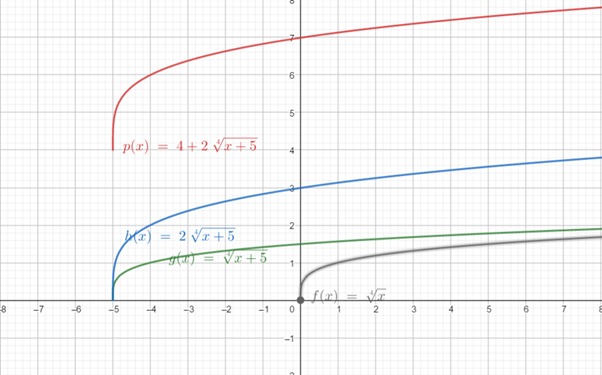# To graph the functionson the same screen and how they related to the graph in part (a). The functions are given below: ( a ) y 1 = x 4 ( b ) y 2 = x + 5 4 ( c ) y 3 = 2 x + 5 4 ( d ) y 4 = 4 + 2 x + 5 4### Precalculus: Mathematics for Calcu...

6th Edition
Stewart + 5 others
Publisher: Cengage Learning
ISBN: 9780840068071### Precalculus: Mathematics for Calcu...

6th Edition
Stewart + 5 others
Publisher: Cengage Learning
ISBN: 9780840068071

#### Solutions

Chapter 2.5, Problem 69E
To determine

## To graph the functionson the same screen and how they related to the graph in part (a).The functions are given below:  (a) y1=x4(b)y2=x+54(c)y3=2x+54(d)y4=4+2x+54

Expert Solution

### Explanation of Solution

Given information:

The function are:

(a) y1=x4(b)y2=x+54(c)y3=2x+54(d)y4=4+2x+54

Graph :

the graph obtained as:Interpretation: from the above graph it is observed that for part(b) shift the graph of part (a) in the left 5 unit.

For part (c) shift the graph of part (a) to the left 5 unit and stretch vertically by a factor of 2 .

For part (d) shift the graph of part (a) to the left 5 unit and stretch vertically by a factor of 2 then shift upward 4 units.

### Have a homework question?

Subscribe to bartleby learn! Ask subject matter experts 30 homework questions each month. Plus, you’ll have access to millions of step-by-step textbook answers!